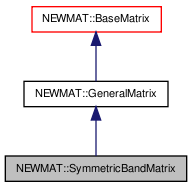# NEWMAT::SymmetricBandMatrix Class Reference

`#include <newmat.h>`

Inheritance diagram for NEWMAT::SymmetricBandMatrix:[legend]

## Detailed Description

Definition at line 1100 of file newmat.h.

List of all members.

## Public Member Functions

SymmetricBandMatrix ()
~SymmetricBandMatrix ()
SymmetricBandMatrix (int n, int lb)
SymmetricBandMatrix (const BaseMatrix &)
void operator= (const BaseMatrix &)
SymmetricBandMatrixoperator= (Real f)
SymmetricBandMatrixoperator= (const SymmetricBandMatrix &m)
Real & operator() (int, int)
Real & element (int, int)
Real operator() (int, int) const
Real element (int, int) const
MatrixType Type () const
SymmetricBandMatrix (const SymmetricBandMatrix &gm)
GeneralMatrixMakeSolver ()
Real SumSquare () const
Real SumAbsoluteValue () const
Real Sum () const
Real MaximumAbsoluteValue () const
Real MinimumAbsoluteValue () const
Real Maximum () const
Real Minimum () const
Real Trace () const
LogAndSign LogDeterminant () const
void GetRow (MatrixRowCol &)
void GetCol (MatrixRowCol &)
void GetCol (MatrixColX &)
void RestoreCol (MatrixRowCol &)
void RestoreCol (MatrixColX &)
GeneralMatrixTranspose (TransposedMatrix *, MatrixType)
void ReSize (int, int)
void ReSize (const GeneralMatrix &A)
bool SameStorageType (const GeneralMatrix &A) const
void ReSizeForAdd (const GeneralMatrix &A, const GeneralMatrix &B)
void ReSizeForSP (const GeneralMatrix &A, const GeneralMatrix &B)
MatrixBandWidth BandWidth () const
void SetParameters (const GeneralMatrix *)
void operator<< (const Real *r)
void operator<< (const int *r)
void operator<< (const BaseMatrix &X)
void swap (SymmetricBandMatrix &gm)

int lower

## Private Member Functions

GeneralMatrixImage () const
void CornerClear () const

## Constructor & Destructor Documentation

 NEWMAT::SymmetricBandMatrix::SymmetricBandMatrix ( )

Definition at line 1107 of file newmat.h.

Referenced by Image().

 NEWMAT::SymmetricBandMatrix::~SymmetricBandMatrix ( )

Definition at line 1108 of file newmat.h.

 NEWMAT::SymmetricBandMatrix::SymmetricBandMatrix ( int n, int lb )

Definition at line 1109 of file newmat.h.

 NEWMAT::SymmetricBandMatrix::SymmetricBandMatrix ( const BaseMatrix & M )

Definition at line 429 of file bandmat.cpp.

 NEWMAT::SymmetricBandMatrix::SymmetricBandMatrix ( const SymmetricBandMatrix & gm )

Definition at line 1123 of file newmat.h.

## Member Function Documentation

 MatrixBandWidth NEWMAT::SymmetricBandMatrix::BandWidth ( ) const` [virtual]`

Reimplemented from NEWMAT::BaseMatrix.

Definition at line 530 of file bandmat.cpp.

 void NEWMAT::SymmetricBandMatrix::CornerClear ( ) const` [private]`

Definition at line 515 of file bandmat.cpp.

Referenced by MaximumAbsoluteValue(), SumSquare(), and SymmetricBandMatrix().

 Real NEWMAT::SymmetricBandMatrix::element ( int m, int n ) const

Definition at line 859 of file newmat6.cpp.

 Real & NEWMAT::SymmetricBandMatrix::element ( int m, int n )

Definition at line 837 of file newmat6.cpp.

 void NEWMAT::SymmetricBandMatrix::GetCol ( MatrixColX & mrc ) ` [virtual]`

Implements NEWMAT::GeneralMatrix.

Definition at line 728 of file newmat3.cpp.

 void NEWMAT::SymmetricBandMatrix::GetCol ( MatrixRowCol & mrc ) ` [virtual]`

Implements NEWMAT::GeneralMatrix.

Definition at line 687 of file newmat3.cpp.

 void NEWMAT::SymmetricBandMatrix::GetRow ( MatrixRowCol & mrc ) ` [virtual]`

Implements NEWMAT::GeneralMatrix.

Definition at line 650 of file newmat3.cpp.

 GeneralMatrix * NEWMAT::SymmetricBandMatrix::Image ( ) const` [private, virtual]`

Reimplemented from NEWMAT::GeneralMatrix.

Definition at line 830 of file newmat4.cpp.

 LogAndSign NEWMAT::SymmetricBandMatrix::LogDeterminant ( ) const` [virtual]`

Reimplemented from NEWMAT::GeneralMatrix.

Definition at line 440 of file bandmat.cpp.

 GeneralMatrix * NEWMAT::SymmetricBandMatrix::MakeSolver ( ) ` [virtual]`

Reimplemented from NEWMAT::GeneralMatrix.

Definition at line 422 of file bandmat.cpp.

 Real NEWMAT::SymmetricBandMatrix::Maximum ( ) const` [virtual]`

Reimplemented from NEWMAT::GeneralMatrix.

Definition at line 1132 of file newmat.h.

 Real NEWMAT::SymmetricBandMatrix::MaximumAbsoluteValue ( ) const` [virtual]`

Reimplemented from NEWMAT::GeneralMatrix.

Definition at line 1128 of file newmat.h.

 Real NEWMAT::SymmetricBandMatrix::Minimum ( ) const` [virtual]`

Reimplemented from NEWMAT::GeneralMatrix.

Definition at line 1133 of file newmat.h.

 Real NEWMAT::SymmetricBandMatrix::MinimumAbsoluteValue ( ) const` [virtual]`

Reimplemented from NEWMAT::GeneralMatrix.

Definition at line 1130 of file newmat.h.

 Real NEWMAT::SymmetricBandMatrix::operator() ( int m, int n ) const

Definition at line 231 of file newmat6.cpp.

 Real & NEWMAT::SymmetricBandMatrix::operator() ( int m, int n )

Definition at line 119 of file newmat6.cpp.

 void NEWMAT::SymmetricBandMatrix::operator<< ( const BaseMatrix & X )

Reimplemented from NEWMAT::GeneralMatrix.

Definition at line 1151 of file newmat.h.

 void NEWMAT::SymmetricBandMatrix::operator<< ( const int * r )

Reimplemented from NEWMAT::GeneralMatrix.

Definition at line 459 of file newmat5.cpp.

 void NEWMAT::SymmetricBandMatrix::operator<< ( const Real * r )

Reimplemented from NEWMAT::GeneralMatrix.

Definition at line 456 of file newmat5.cpp.

Referenced by operator<<().

 SymmetricBandMatrix& NEWMAT::SymmetricBandMatrix::operator= ( const SymmetricBandMatrix & m )

Definition at line 1113 of file newmat.h.

 SymmetricBandMatrix& NEWMAT::SymmetricBandMatrix::operator= ( Real f )

Reimplemented from NEWMAT::GeneralMatrix.

Definition at line 1112 of file newmat.h.

 void NEWMAT::SymmetricBandMatrix::operator= ( const BaseMatrix & X )

Definition at line 508 of file bandmat.cpp.

Referenced by operator=().

 void NEWMAT::SymmetricBandMatrix::ReSize ( const GeneralMatrix & A ) ` [virtual]`

Reimplemented from NEWMAT::GeneralMatrix.

Definition at line 458 of file bandmat.cpp.

 void NEWMAT::SymmetricBandMatrix::ReSize ( int n, int lb )

Definition at line 449 of file bandmat.cpp.

Referenced by SymmetricBandMatrix().

 void NEWMAT::SymmetricBandMatrix::ReSizeForAdd ( const GeneralMatrix & A, const GeneralMatrix & B ) ` [virtual]`

Reimplemented from NEWMAT::GeneralMatrix.

Definition at line 483 of file bandmat.cpp.

 void NEWMAT::SymmetricBandMatrix::ReSizeForSP ( const GeneralMatrix & A, const GeneralMatrix & B ) ` [virtual]`

Reimplemented from NEWMAT::GeneralMatrix.

Definition at line 495 of file bandmat.cpp.

 void NEWMAT::SymmetricBandMatrix::RestoreCol ( MatrixColX & mrc ) ` [virtual]`

Reimplemented from NEWMAT::GeneralMatrix.

Definition at line 773 of file newmat3.cpp.

 void NEWMAT::SymmetricBandMatrix::RestoreCol ( MatrixRowCol & ) ` [virtual]`

Reimplemented from NEWMAT::GeneralMatrix.

Definition at line 1139 of file newmat.h.

 bool NEWMAT::SymmetricBandMatrix::SameStorageType ( const GeneralMatrix & A ) const` [virtual]`

Reimplemented from NEWMAT::GeneralMatrix.

Definition at line 476 of file bandmat.cpp.

 void NEWMAT::SymmetricBandMatrix::SetParameters ( const GeneralMatrix * gmx ) ` [virtual]`

Reimplemented from NEWMAT::GeneralMatrix.

Definition at line 446 of file bandmat.cpp.

 short NEWMAT::SymmetricBandMatrix::SimpleAddOK ( const GeneralMatrix * gm ) ` [private, virtual]`

Reimplemented from NEWMAT::GeneralMatrix.

Definition at line 72 of file bandmat.cpp.

 Real NEWMAT::SymmetricBandMatrix::Sum ( ) const` [virtual]`

Reimplemented from NEWMAT::GeneralMatrix.

Definition at line 556 of file bandmat.cpp.

 Real NEWMAT::SymmetricBandMatrix::SumAbsoluteValue ( ) const` [virtual]`

Reimplemented from NEWMAT::GeneralMatrix.

Definition at line 546 of file bandmat.cpp.

 Real NEWMAT::SymmetricBandMatrix::SumSquare ( ) const` [virtual]`

Reimplemented from NEWMAT::GeneralMatrix.

Definition at line 536 of file bandmat.cpp.

 void NEWMAT::SymmetricBandMatrix::swap ( SymmetricBandMatrix & gm )

Definition at line 1077 of file newmat4.cpp.

Referenced by NEWMAT::swap().

 Real NEWMAT::SymmetricBandMatrix::Trace ( ) const` [virtual]`

Reimplemented from NEWMAT::BaseMatrix.

Definition at line 594 of file newmat8.cpp.

 GeneralMatrix * NEWMAT::SymmetricBandMatrix::Transpose ( TransposedMatrix * , MatrixType mt ) ` [virtual]`

Reimplemented from NEWMAT::GeneralMatrix.

Definition at line 437 of file bandmat.cpp.

 MatrixType NEWMAT::SymmetricBandMatrix::Type ( ) const` [virtual]`

Implements NEWMAT::GeneralMatrix.

Definition at line 438 of file newmat4.cpp.

## Member Data Documentation

Definition at line 1106 of file newmat.h.

Referenced by element(), GetCol(), GetRow(), operator()(), RestoreCol(), swap(), and Trace().

The documentation for this class was generated from the following files:

 newmat11b Generated Mon May 9 04:54:19 2016 by Doxygen 1.6.3